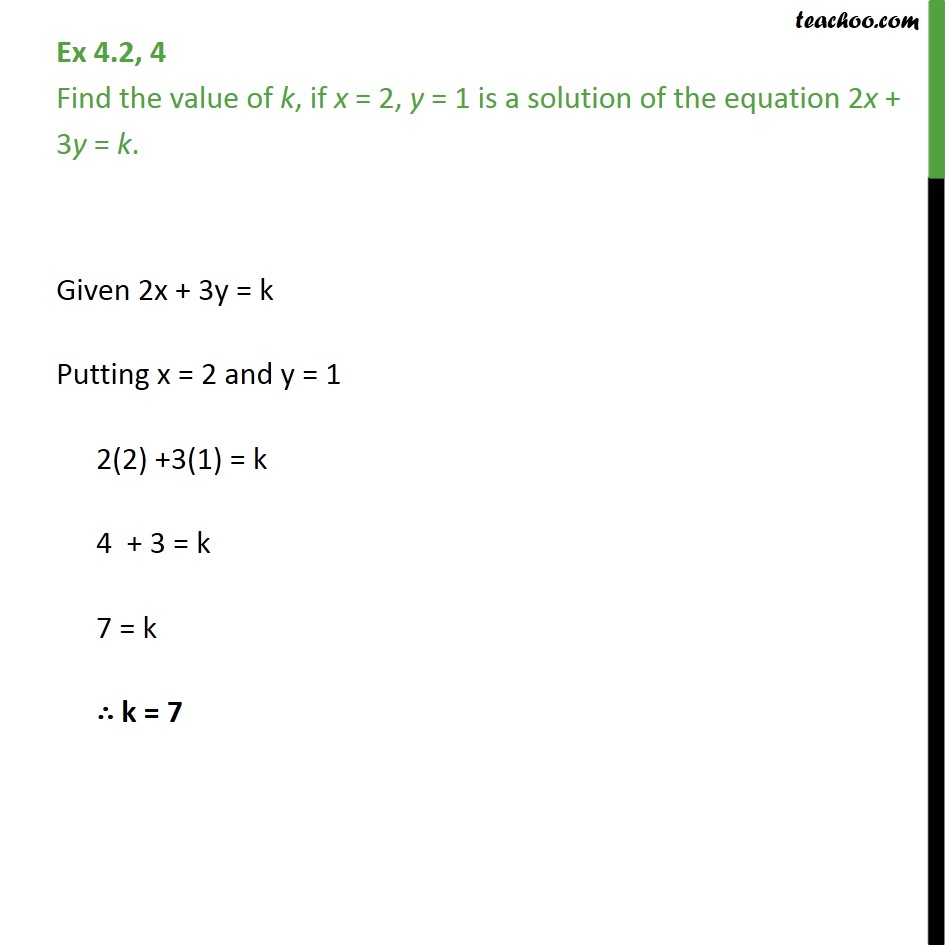Subscribe to our Youtube Channel - https://you.tube/teachoo

1. Class 9
2. Important Questions for Exam - Class 9
3. Chapter 4 Class 9 Linear Equations in Two Variables

Transcript

Ex 4.2, 4 - Chapter 4 Class 9 Linear Equations in Two Variables - NCERT Find the value of k, if x = 2, y = 1 is a solution of the equation 2x + 3y = k. Given 2x + 3y = k Putting x = 2 and y = 1 2(2) +3(1) = k 4 + 3 = k 7 = k ∴ k = 7

Chapter 4 Class 9 Linear Equations in Two Variables

Class 9
Important Questions for Exam - Class 9